Mechanics

# Work done by various forces

Work is the energy spent on an activity. Acting together, several forces can perform it on a body.

In physics, the definition of work is very different from the everyday definition. In our daily life, we associate the word work with the fact that we carry out some task, such as washing dishes, sweeping the yard, mowing the lawn, etc.

When we push a car, for example, for a certain distance, it is necessary to exert force in order to overcome the friction force all the way. In Physics, we say that we are doing work on the mobile, that is, on the car, to move it from an initial point to an end point. If we think about it, we’ll have to do twice as much work as we did in the initial situation to move the car twice as long as the first one.

In Physics , therefore, we define the work done by a force as the product of the force and displacement. Since force and displacement are vector quantities , work is defined as the dot product of force and displacement . Mathematically, we have: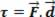Work is a scalar quantity obtained by the dot product of two vectors. In the international system of units ( SI ) , the unit of measure of work is the joule ( J ).

And when we no longer have one force doing the work, but several forces? How is it possible to determine the work done by these forces?

In the case where several forces act on a body, we determine the work done by the forces by summing up all the work done by each of these forces. In this situation, we can find two different ways to perform the calculation of the work done. Let’s see what they are:

In the first way, we can determine the work done by the various forces by finding the resultant force acting on the body and calculating the work of the resultant force.

In the second way, we can sum the work of each of the forces independently of the others.

The first way can be determined as a function of the resultant force and displacement.

Mathematically, we have: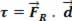Where R is the resultant force acting on the body.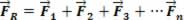Which is equivalent to performing the sum of the work done by each force separately: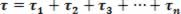Or yet: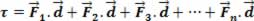We can verify that both ways allow us to obtain as a result the same value for the total work done by various forces.